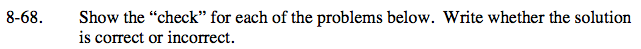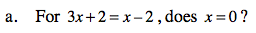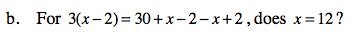Home > ACC7 > Chapter cc38 > Lesson cc38.2.2 > Problem8-68

8-68.
1. Show the “check” for each of the problems below. Write whether the solution is correct or incorrect. Homework Help ✎

1. For 3x + 2 = x − 2, does x = 0?

2. For 3(x − 2) = 30 + x − 2 − x + 2, does x = 12?Substitute 0 for x.

3 (0) + 2 = (0) − 2
Now simplify.

0 + 2 = 0 − 2

2 = −2

Is this statement true?Follow the steps in part (a).